# Product of two fractions

Product of two fractions is 9 3/5 . If one of the fraction is 9 3/7. Find the other fraction.

a =  1.0182

### Step-by-step explanation: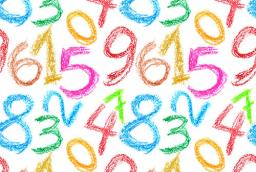Did you find an error or inaccuracy? Feel free to write us. Thank you!Tips to related online calculators
Need help to calculate sum, simplify or multiply fractions? Try our fraction calculator.

## Related math problems and questions:

• Division by zero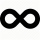Fraction 5 by 2. if 3 is added to numerator and 2 is subtracted from the denominator then the new fraction is:
• Two numbers and its productThe product of two numbers are 2/3. If on of them is 1/10, what is the other?
• FractionFind for what x fraction (-4x -6)/(x) equals:
• What fraction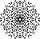What fraction of number 1 to 30 is prime?
• Quotient and productIf the quotient of [8/5 divided by 8/10] is added to the product of [8/14 x 7/12 x 3/8], what is the sum?
• Without 2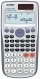Without multipying, tell whether the product 0.644 x 0.25 will be greater than 1 or less than 1? Explane how you know. Then find the product.
• JerryJerry has 3/4 of a pizza. He needs to share it with 6 friends. What fraction of the pizza will each friend get? Only write the fraction
• Divide 11Divide the product of 4 and 5/8 by 1 1/2. Write your answer as a mixed number.
• Fraction unknowns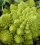Divide of fractions with unknowns: Fraction 1: The quantity x squared plus 6 times x plus 9 over the quantity x minus 1. Fraction 2: the quantity x squared minus 9 over the quantity x squared minus 2 times x plus 1.  Find Fraction 1 over Fraction 2.
• A quotientWhat is the quotient of 3/10 divided by 2/4 as a fraction?
• Write 3Write a real world problem involving the multiplication of a fraction and a whole number with a product that is between 8 and 10 then solve the problem
• Product increased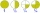When the product of 2/3 and 6/10 is increased by 2/5, the result is?
• Quotient 3If the quotient of 8/13 and 2 is subtracted from the product of 1 3/4 and 8/21, what is the difference?
• Divide 13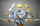Divide. Simplify your answer and write as an improper fraction or whole number. 14÷8/3
• Mixed numbersRewrite mixed numbers, so the fractions have the same denominator: 5 1/5 - 2 2/3
• Quotient and divisionFind the quotient of 3/4 and 1/4.
• EvaluateThe division of numbers $a and$b increase by-product of the numbers $c and$d